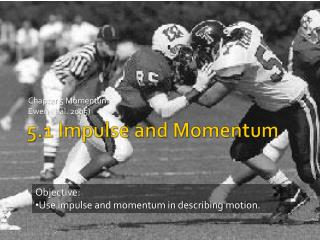Download Presentation5.1 Impulse and Momentum

# 5.1 Impulse and Momentum

Download Presentation## 5.1 Impulse and Momentum

- - - - - - - - - - - - - - - - - - - - - - - - - - - E N D - - - - - - - - - - - - - - - - - - - - - - - - - - -
##### Presentation Transcript

1. Chapter 5 Momentum Ewen et al. 2005) 5.1 Impulse and Momentum • Objective: • Use impulse and momentum in describing motion.

2. Momentum • Is a measure of the amount of inertia and motion and object has. • Another way to think about momentum is how difficult it is to bring a moving object to rest. • It is the product of mass and velocity. • Units? • kgm/s and slugft/s • Momentum is a vector quantity.

3. Example 1 • Find the momentum of an auto with mass 105 slugs traveling 60.0 mi/h.

4. Example 2 • Find the momentum of an auto with mass 1350 kg traveling 75.0 km/h.

5. Example 3 • Find the velocity a bullet of mass 1.00 x 10-2 kg would have to have so that it has the same momentum as a lighter bullet of mass 1.80 x 10-3 kg and velocity of 325 m/s. Problems 5.1: Do problems 1-11, odd.

6. Impulse • Impulse on an object is the product of the force and the time interval during which the force acts on the object. • How are impulse and momentum related? • Recall that and

7. Combining Eq. 1 and Eq. 2 • Then, distributing m • Multiply both sides by t • Thus impulse (Ft) is equal to final minus initial momentums (mv), i.e. change in momentum.

8. After this analysis, we see that impulse is the measure of the change in momentum of an object in response to an exerted force. • Some interesting outcomes • Given enough time and distance, any force no matter how small can bring the largest object to p = 0 when given enough time. • The importance of “followthough” in sports.

9. Example 4 • A 17.5-g bullet is fired at a muzzle velocity of 582 m/s from a gun with a mass of 8.00 kg and a barrel length of 75.0 cm. • How long is the bullet in the barrel? • What is the force on the bullet while it is in the barrel? • Find the impulse exerted on the bullet while it is in the barrel. • Find the bullet’s momentum as it leaves the barrel.

10. Law of Conservation of Momentum • When no outside forces are acting on a system of moving objects, the total momentum of a system remains constant.

11. Example of Conservation of Momentum Both at Rest After Push vboy = -0.40 m/s vman = ? mman = 75 kg mboy = 35 kg

12. Impulse-Momentum Theorem

13. Example 5 • What force is required to slow a 1450 kg care traveling 115 km/h to 45.0 km/h within 3.00s? How far does the car travel during its deceleration?